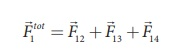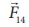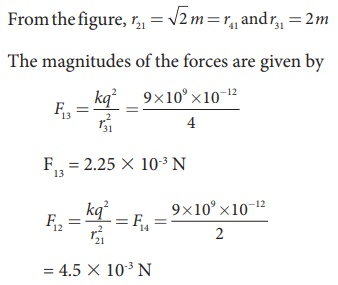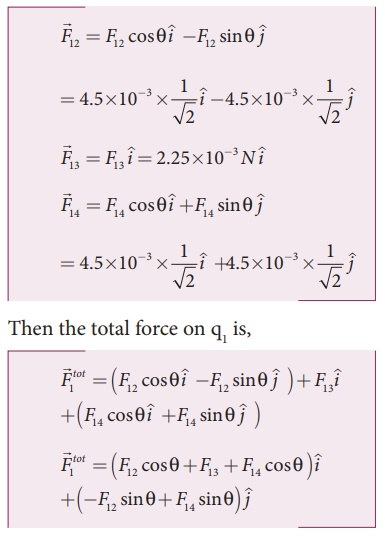Home | | Physics 12th Std | Coulomb’s Law: Superposition principle

# Coulomb’s Law: Superposition principle

Coulomb’s law explains the interaction between two point charges.

Superposition principle

Coulomb’s law explains the interaction between two point charges. If there are more than two charges, the force on one charge due to all the other charges needs to be calculated. Coulomb’s law alone does not give the answer. The superposition principle explains the interaction between multiple charges.

According to this superposition principle, the total force acting on a given charge is equal to the vector sum of forces exerted on it by all the other charges.

Consider a system of n charges, namely q1, q2, q3 ….qn. The force on q1 exerted by the charge q2Here ^r21 is the unit vector from q2 to q1 along the line joining the two charges and r21 is the distance between the charges q1 and q2. The electrostatic force between two charges is not affected by the presence of other charges in the neighbourhood.

The force on q1 exerted by the charge q3 isBy continuing this, the total force acting on the charge q1 due to all other charges is given by### EXAMPLE 1.5

Consider four equal charges q1,q2, q3 and q4 = q = +1μC located at four different points on a circle of radius 1m, as shown in the figure. Calculate the total force acting on the charge q1 due to all the other charges.### Solution

According to the superposition principle, the total electrostatic force on charge q1 is the vector sum of the forces due to the other charges,The following diagram shows the direction of each force on the charge q1.The charges q2 and q4 are equi-distant from q1. As a result the strengths (magnitude) of the forcesandare the same even though their directions are different. Therefore the vectors representing these two forces are drawn with equal lengths. But the charge q3 is located farther compared to q2 and q4. Since the strength of the electrostatic force decreases as distance increases, the strength of the forceis lesser than that of forcesand. Hence the vector representing the forceis drawn with smaller length compared to that for forcesand.From the figure, the angle θ = 45º. In terms of the components, we haveSince F12 = F14, the jth component is zero.

Hence we havesubstituting the values in the above equation,The resultant force is along the positive x axis.

Tags : Electrostatics , 12th Physics : Electrostatics
Study Material, Lecturing Notes, Assignment, Reference, Wiki description explanation, brief detail
12th Physics : Electrostatics : Coulomb’s Law: Superposition principle | Electrostatics